# 自然语言处理（NLP）讲义

## 一、NLP概述

### 1. NLP的定义

NLP（Nature Language Processing，自然语言处理）是计算机学科及人工智能领域一个重要的子学科，它主要研究计算机如何处理、理解及应用人类语言。所谓自然语言，指人说的话、人写的文章，是人类在长期进化过程中形成的一套复杂的符号系统（类似于C/Java等计算机语言则称为人造语言）。以下是关于自然语言处理常见的定义：

• 自然语言处理是计算机科学与语言中关于计算机与人类语言转换的领域。——中文维基百科
• 自然语言处理是人工智能领域中一个重要的方向。它研究实现人与计算机之间用自然语言进行有效沟通的各种理论和方法。——百度百科
• 自然语言处理研究在人与人交际中及人与计算机交际中的语言问题的一门学科。NLP要研制表示语言能力和语言应用的模型，建立计算机框架来实现这些语言模型，提出相应的方法来不断完善这种模型，并根据语言模型设计各种实用系统，以及对这些系统的评测技术。——Bill Manaris，《从人机交互的角度看自然语言处理》

### 2. NLP的主要任务

NLP的主要任务可以分为两大类，一类是基于现有文本或语料的分析，另一类是生成新的文本或语料。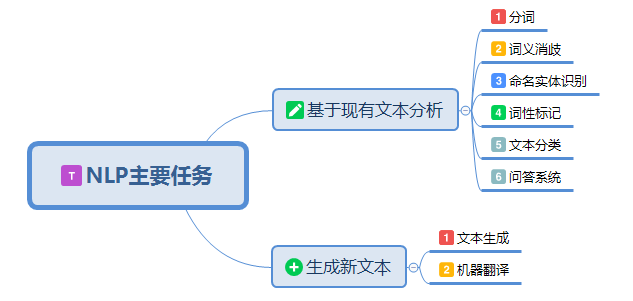#### 3）命名实体识别（NER）

NER尝试从给定的文本主体或文本语料库中提取实体（例如，人物、位置和组织）。例如，句子：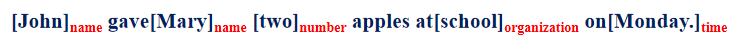#### 4）词性标记（PoS）

PoS标记是将单词分配到各自对应词性的任务。它既可以是名词、动词、形容词、副词、介词等基本词、也可以是专有名词、普通名词、短语动词、动词等。

#### 5）问答（QA）系统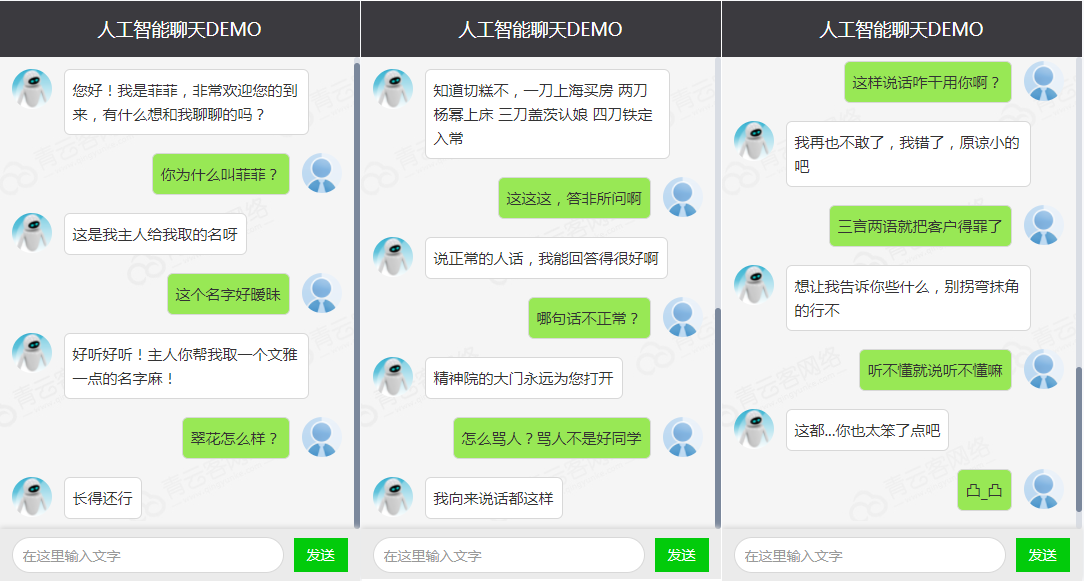#### 6）机器翻译（MT）### 3. NLP的发展历程

NLP的发展轨迹为：基于规则 → 基于统计 → 基于深度学习，其发展大致经历了4个阶段：1956年以前的萌芽期；19571970年的快速发展期；19711993年的低速发展期；1994年至今的复苏融合期。

#### 1）萌芽期（1956年以前）

• 1946年：第一台电子计算机诞生
• 1948年：Shannon把离散马尔可夫过程的概率模型应用于描述语言的自动机。接着，他又把热力学中“熵”(entropy)的概念引用于语言处理的概率算法中
• 1956年：Chomsky又提出了上下文无关语法，并把它运用到自然语言处理中

#### 4）复苏融合期（1994年至今）

90年代中期以后，有两件事从根本上促进了自然语言处理研究的复苏与发展。一件事是90年代中期以来，计算机的速度和存储量大幅增加，为自然语言处理改善了物质基础，使得语音和语言处理的商品化开发成为可能；另一件事是1994年Internet商业化和同期网络技术的发展使得基于自然语言的信息检索和信息抽取的需求变得更加突出。以下列举除了2000年之后NLP领域的几个里程碑事件：

• 2001年：神经语言模型

• 2008年：多任务学习

• 2013年： Word嵌入

• 2013年：NLP的神经网络

• 2014年：序列到序列模型

• 2015年：注意力机制

• 2015年：基于记忆的神经网络

• 2018年：预训练语言模型

### 4. NLP的困难与挑战

#### 1）语言歧义

“保护”理解成动词、名词，语义不一样

#### 2）不同语言结构差异### 5. NLP相关知识构成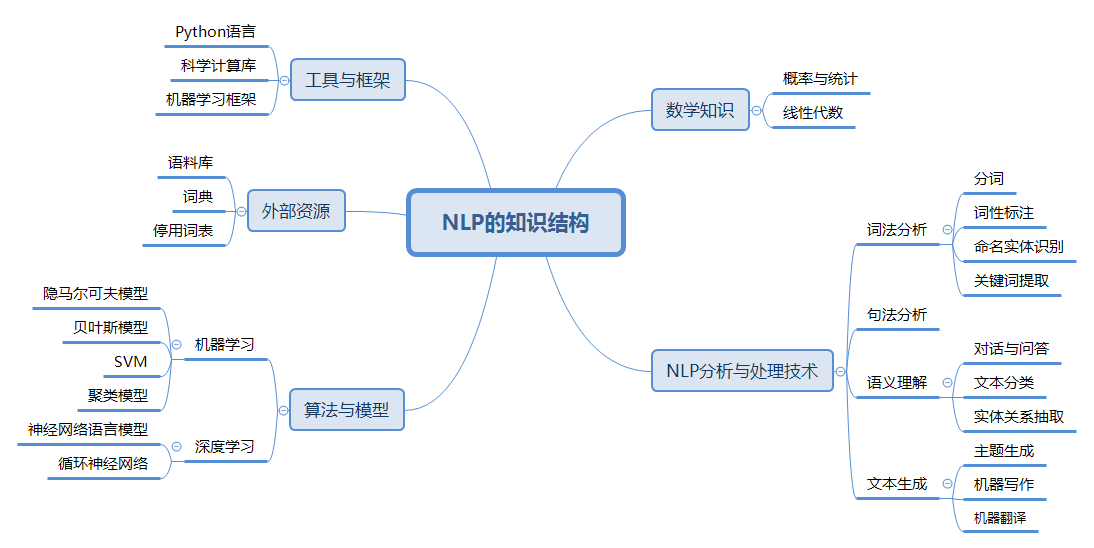### 6. 语料库

#### 2）语料库的特征

• 语料库中存放的是实际中真实出现过的语言材料
• 语料库是以计算机为载体承载语言知识的基础资源，但不等于语言知识
• 真实语料需要经过分析、处理和加工，才能成为有用的资源

#### 3）语料库的作用

• 支持语言学研究和语言教学研究
• 支持NLP系统的开发

## 二、传统NLP处理技术

### 1. 中文分词

#### 1）正向最大匹配法

• 从左向右取待分汉语句的m个字作为匹配字段，m为词典中最长词的长度；
• 查找词典进行匹配；
• 若匹配成功，则将该字段作为一个词切分出去；
• 若匹配不成功，则将该字段最后一个字去掉，剩下的字作为新匹配字段，进行再次匹配；
• 重复上述过程，直到切分所有词为止。

#### 3）双向最大匹配法

• 如果正反向分词结果词数不同，则取分词数量少的那个；

• 分词结果相同，没有歧义，返回任意一个；分词结果不同，返回其中单字数量较少的那个。

【示例1】正向最大匹配分词法

【示例2】逆向最大匹配分词法

【示例3】Jieba库分词

Jieba是一款开源的、功能丰富、使用简单的中文分词工具库，它提供了三种分词模式：

• 精确模式：试图将句子最精确地分词，适合文本分析
• 全模式：把句子中所有可以成词的词语分割出来，速度快，但有重复词和歧义
• 搜索引擎模式：在精确模式基础上，对长词再次切分，提高召回率，适合用于搜索引擎分词

【示例4】文本高频词汇提取

### 2. 词性标注

#### 3）词性标注规范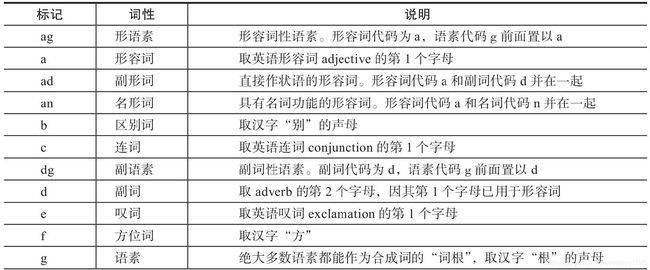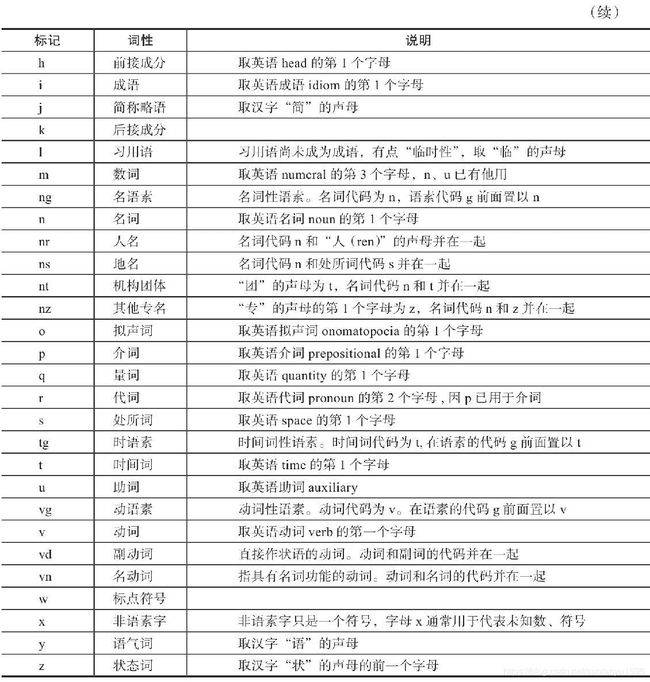#### 4）Jieba库词性标注

Jieba库提供了词性标注功能，采用结合规则和统计的方式，具体为在词性标注的过程中，词典匹配和HMM共同作用。词性标注流程如下：

【示例】Jieba库实现词性标注

### 3. 命名实体识别（NER）

（1）各类命名实体的数量众多。

（2）命名实体的构成规律复杂。

（2）嵌套情况复杂。

（4）长度不确定。

（1）基于规则的命名实体识别。规则加词典是早期命名实体识别中最行之有效的方式。其依赖手工规则的系统，结合命名实体库，对每条规则进行权重赋值，然后通过实体与规则的相符情况来进行类型判断。这种方式可移植性差、更新维护困难等问题。

（2）基于统计的命名实体识别。基于统计的命名实体识别方法有：隐马尔可夫模型、最大熵模型、条件随机场等。其主要思想是基于人工标注的语料，将命名实体识别任务作为序列标注问题来解决。基于统计的方法对语料库的依赖比较大，而可以用来建设和评估命名实体识别系统的大规模通用语料库又比较少，这是该方法的一大制约。

（3）基于深度学习的方法。利用深度学习模型，预测词（或字）是否为命名实体，并预测出起始、结束位置。

（4）混合方法。将前面介绍的方法混合使用。

### 4. 关键词提取

#### 1）TF-IDF算法

TF-IDF（Term Frequency-Inverse Document Frequency，词频-逆文档频率）是一种基于传统的统计计算方法，常用于评估一个文档集中一个词对某份文档的重要程度。其基本思想是：一个词语在文档中出现的次数越多、出现的文档越少，语义贡献度越大（对文档区分能力越强）。TF-IDF表达式由两部分构成，词频、逆文档频率。词频定义为：

$TF_{ij} = \frac{n_{ji}}{\sum_k n_{kj}}$

$IDF_i = log(\frac{|D|}{|D_i| + 1})$

$TF-IDF = TF_{ij} \times IDF_i =\frac{n_{ji}}{\sum_k n_{kj}} \times log(\frac{|D|}{|D_i| + 1})$

$TF_{献血} = 2 / 30 \approx 0.067 \\ TF_{血液} = 2 / 30 \approx 0.067 \\ TF_{进行} = 2 / 30 \approx 0.067 \\ TF_{公示} = 2 / 30 \approx 0.067$

$TF-IDF_{献血} = 0.067 * log(1000/10) = 0.067 * 2 = 0.134\\ TF-IDF_{血液} = 0.067 * log(1000/15) = 0.067 * 1.824 = 0.1222 \\ TF-IDF_{进行} = 0.067 * log(1000/100) = 0.067 * 1 = 0.067 \\ TF-IDF_{公示} = 0.067 * log(1000/50) = 0.067 * 1.30 = 0.08717$

“献血”、“血液”的TF-IDF值最高，所以为最适合这篇文档的关键词。

#### 2）TextRank算法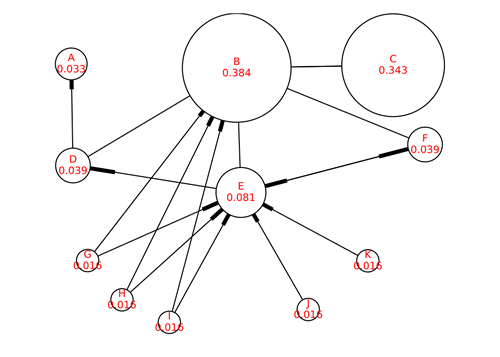PageRank基本思想有两条：

• 链接数量。一个网页被越多的其它网页链接，说明这个网页越重要
• 链接质量。一个网页被一个越高权值的网页链接，也能表名这个网页越重要

$S(V_i) = \sum_{j \in In(V_i)} \Bigg( \frac{1}{Out(V_j)} \times S(V_j) \Bigg)$

$S(V_i) = (1 - d) + d \times \sum_{j \in In(V_i)} \Bigg( \frac{1}{Out(V_j)} \times S(V_j) \Bigg)$

### 6. 综合案例

#### 1）垃圾邮件分类

• 数据集介绍：包含5000份正常邮件、5001份垃圾邮件的样本
• 文本特征处理方式：采用TF-IDF作为文本特征值
• 模型选择：朴素贝叶斯、支持向量机模型
• 基本流程：读取数据 → 去除停用词和特殊符号 → 计算TF-IDF特征值 → 模型训练 → 预测 → 打印结果

## 三、文本表示

### 1. One-hot

One-hot（独热）编码是一种最简单的文本表示方式。如果有一个大小为V的词表，对于第i个词$w_i$，可以用一个长度为V的向量来表示，其中第i个元素为1，其它为0.例如：

One-hot词向量构建简单，但也存在明显的弱点：

• 维度过高。如果词数量较多，每个词需要使用更长的向量表示，造成维度灾难；
• 稀疏矩阵。每个词向量，其中只有一位为1，其它位均为零；
• 语义鸿沟。词语之间的相似度、相关程度无法度量。

### 2. 词袋模型

• 丢失了顺序和语义。顺序是极其重要的语义信息，词袋模型只统计词语出现的频率，忽略了词语的顺序。例如上述两个句子意思相反，但词袋模型表示却完全一致；
• 高维度和稀疏性。当语料增加时，词袋模型维度也会增加，需要更长的向量来表示。但大多数词语不会出现在一个文本中，所以导致矩阵稀疏。

### 3. TF-IDF

TF-IDF（Term Frequency-Inverse Document Frequency，词频-逆文档频率）是一种基于传统的统计计算方法，常用于评估一个文档集中一个词对某份文档的重要程度。其基本思想是：一个词语在文档中出现的次数越多、出现的文档越少，语义贡献度越大（对文档区分能力越强）。其表达式为：

$TF-IDF = TF_{ij} \times IDF_i =\frac{n_{ji}}{\sum_k n_{kj}} \times log(\frac{|D|}{|D_i| + 1})$

### 4. 共现矩阵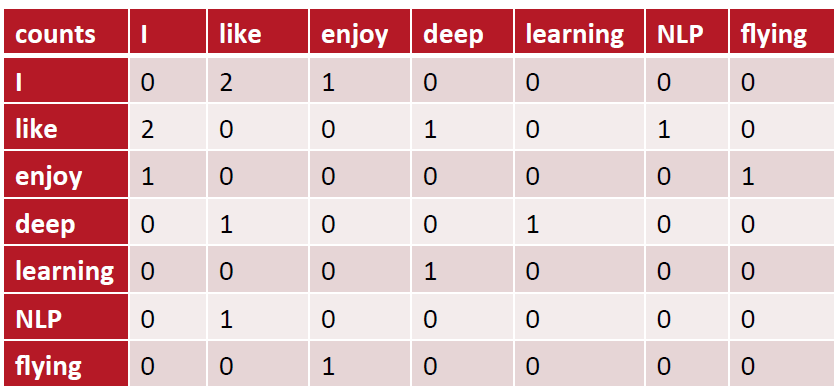### 5. N-Gram表示

N-Gram模型是一种基于统计语言模型，语言模型是一个基于概率的判别模型，它的输入是个句子（由词构成的顺序序列），输出是这句话的概率，即这些单词的联合概率。

N-Gram本身也指一个由N个单词组成的集合，各单词具有先后顺序，且不要求单词之间互不相同。常用的有Bi-gram（N=2）和Tri-gram（N=3）。例如：

Bi-gram: {I, love}, {love, deep}, {deep, learning}

Tri-gram: {I, love, deep}, {love deep learning}

N-Gram基本思想是将文本里面的内容按照字节进行大小为n的滑动窗口操作，形成了长度是n的字节片段序列。每一个字节片段称为一个gram，对所有gram的出现频度进行统计，并按照事先设置好的频度阈值进行过滤，形成关键gram列表，也就是这个文本向量的特征空间，列表中的每一种gram就是一个特征向量维度。

### 6. 词嵌入

#### 1）什么是词嵌入

Man Women King Queen Apple Orange
Gender -1 1 -0.95 0.97 0.00 0.01
Royal 0.01 0.02 0.93 0.95 -0.01 0.00
Age 0.03 0.02 0.70 0.69 0.03 -0.02
Food 0.09 0.01 0.02 0.01 0.95 0.97

$e_{man} - e_{woman} = \left[ \begin{matrix} -1 \\ 0.01 \\ 0.03 \\ 0.09 \\ \end{matrix} \right] -\left[ \begin{matrix} 1 \\ 0.02 \\ 0.02 \\ 0.01 \\ \end{matrix} \right] = \left[ \begin{matrix} -2 \\ -0.01 \\ 0.01 \\ 0.08 \\ \end{matrix} \right] \approx \left[ \begin{matrix} -2 \\ 0 \\ 0 \\ 0 \\ \end{matrix} \right]$

$e_{man} - e_{woman} = \left[ \begin{matrix} -0.95 \\ 0.93 \\ 0.70 \\ 0.02 \\ \end{matrix} \right] -\left[ \begin{matrix} 0.97 \\ 0.85 \\ 0.69 \\ 0.01 \\ \end{matrix} \right] = \left[ \begin{matrix} -1.92 \\ -0.02 \\ 0.01 \\ 0.01 \\ \end{matrix} \right] \approx \left[ \begin{matrix} -2 \\ 0 \\ 0 \\ 0 \\ \end{matrix} \right]$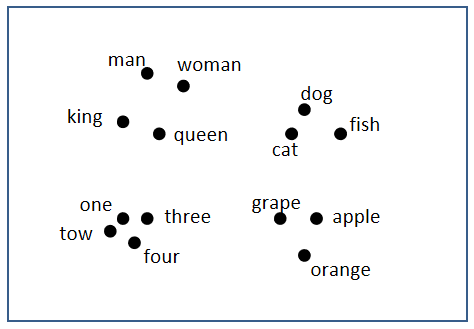#### 2）词嵌入的优点

• 特征稠密；
• 能够表征词与词之间的相似度；
• 泛化能力更好，支持语义计算。

## 四、语言模型

### 1. 什么是语言模型

$P(w_1, w_2, ..., w_n) = P(w_1)P(w_2|w_1)P(w_3|w_1,w_2)...P(w_m|w_1,w_2,...,w_{m-1})$

### 2. N-gram模型

$P(w_1, w_2, ..., w_n) = \prod_{i=1}^m P(w_i)$

$P(w_1, w_2, ..., w_n) = \prod_{i=1}^m P(w_i|w_{i-1})$

$P(w_1, w_2, ..., w_n) = \prod_{i=1}^m P(w_i|w_{i-2}, w_{i-1})$

### 3. 神经网络语言模型（NNLM）

NNLM是利用神经网络对N元条件进行概率估计的一种方法，其基本结构如下图所示：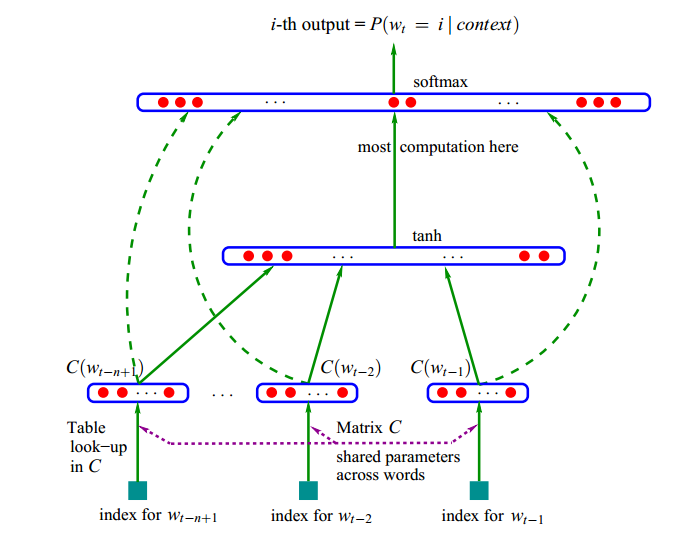• 输入：前N-1个词语的向量

• 输出：第N个词语的一组概率

• 目标函数：

$f(w_t, t_{t-1}, ..., w_{t-n+1}) = p(p_t|w_1^{t-1})$

• 特征映射：将输入映射为一个特征向量，映射矩阵$C \in R^{|V| \times m}$
• 计算条件概率分布：通过另一个函数，将特征向量转化为一个概率分布

$h = tanh(Hx + b) \\ y = Uh + d$

H为隐藏层权重矩阵，U为隐藏层到输出层的权重矩阵。输出层加入softmax函数，将y转换为对应的概率。模型参数$\theta$，包括：

$\theta = (b, d, H, U, C)$

### 4. Word2vec

Word2vec是Goolge发布的、应用最广泛的词嵌入表示学习技术，其主要作用是高效获取词语的词向量，目前被用作许多NLP任务的特征工程。Word2vec 可以根据给定的语料库，通过优化后的训练模型快速有效地将一个词语表达成向量形式，为自然语言处理领域的应用研究提供了新的工具，包含Skip-gram（跳字模型）和CBOW（连续词袋模型）来建立词语的词嵌入表示。Skip-gram的主要作用是根据当前词，预测背景词（前后的词）；CBOW的主要作用是根据背景词（前后的词）预测当前词。

#### 1）Skip-gram

Skip-gram的主要作用是根据当前词，预测背景词（前后的词），其结构图如下图所示：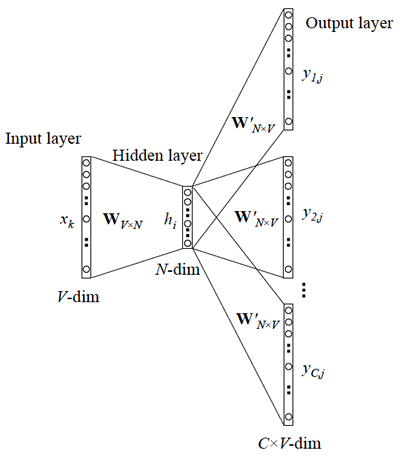$Text = {w_1, w_2, w_3, ..., w_n}$

$argmax \prod_{c \in Text} \prod_{c \in c(w)} P(c|w; \theta)$

$argmax \sum_{c \in Text} \sum_{c \in c(w)} logP(c|w;\theta)$

$argmax \sum_{c \in Text} \sum_{c \in c(w)} log \frac{e^{u_c \cdot v_w}}{\sum_{c' \in vocab }e_{c'} \cdot v_w}$

$argmax \sum_{c \in Text} \sum_{c \in c(w)} u_c \cdot v_w - log \sum_{c' \in vocab }e_{c'} \cdot v_w$

$argmax (\prod_{w,c \in D} log P(D=1|w,c; \theta) \prod_{w, c \in D'} P(D=0|w, c; \theta)) \\ = argmax (\prod_{w,c \in D} \frac{1}{1+exp(-U_c \cdot V_w)} \prod_{w, c \in D'} [1- \frac{1}{1+exp(-U_c \cdot V_w)}]) \\ = argmax(\sum_{w,c \in D} log \sigma (U_c \cdot V_w) + \sum_{w,c \in D'} log \sigma (-U_c \cdot V_w))$

#### 2）CBOW模型

CBOW模型全程为Continous Bag of Words（连续词袋模型），其核心思想是用上下文来预测中心词，例如：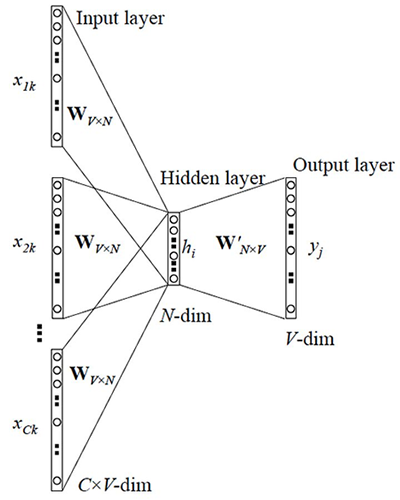• 输入：$C \times V$的矩阵，C表示上下文词语的个数，V表示词表大小
• 隐藏层：$V \times N$的权重矩阵，一般称为word-embedding，N表示每个词的向量长度，和输入矩阵相乘得到$C \times N$的矩阵。综合考虑上下文中所有词信息预测中心词，所以将$C \times N$矩阵叠加，得到$1 \times N$的向量
• 输出层：包含一个$N \times V$的权重矩阵，隐藏层向量和该矩阵相乘，输出$1 \times V$的向量，经过softmax转换为概率，对应每个词表中词语的概率

#### 3）示例：训练词向量

• 安装gensim
• 用于解析XML，读取XML文件中的数据，并写入到新的文本文件中
• 生成分词文件
• 训练
• 测试

### 5. 循环神经网络（RNN）

#### 1）原生RNN

##### ① RNN起源及发展

1982年，物理学家约翰·霍普菲尔德（John Hopfield）利用电阻、电容和运算放大器等元件组成的模拟电路实现了对网络神经元的描述，该网络从输出到输入有反馈连接。1986年，迈克尔·乔丹（Michael Jordan，不是打篮球那哥们，而是著名人工智能学者、美国科学院院士、吴恩达的导师）借鉴了Hopfield网络的思想，正式将循环连接拓扑结构引入神经网络。1990年，杰弗里·埃尔曼（Jeffrey Elman）又在Jordan的研究基础上做了部分简化，正式提出了RNN模型（那时还叫Simple Recurrent Network，SRN）。

##### ② RNN的结构

RNN结构如下图所示：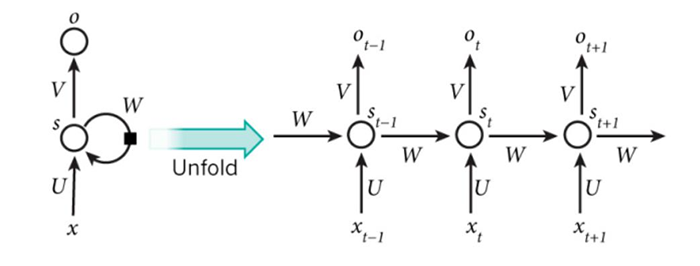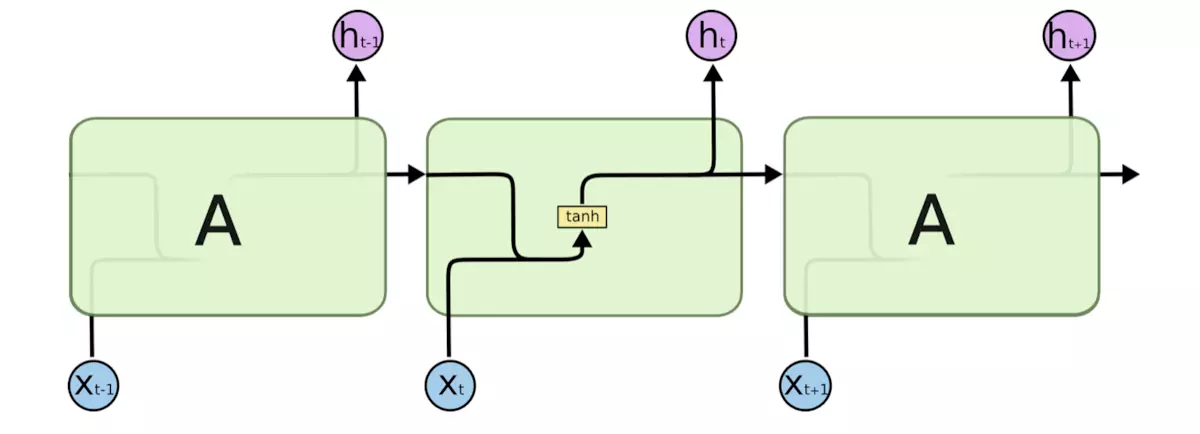$s_t = f(U \cdot x_t + W \cdot s_{t-1} + b) \\ y_t = g(V \cdot s_t + d)$

##### ③ RNN的功能

RNN善于处理跟序列相关的信息，如：语音识别，语言建模，翻译，图像字幕。它能根据近期的一些信息来执行/判别/预测当前任务。例如：

#### 2）长短期记忆模型（LSTM）

LSTM同样具有链式结构，它具有4个以特殊方式互相影响的神经网络层。其结构入下图所示：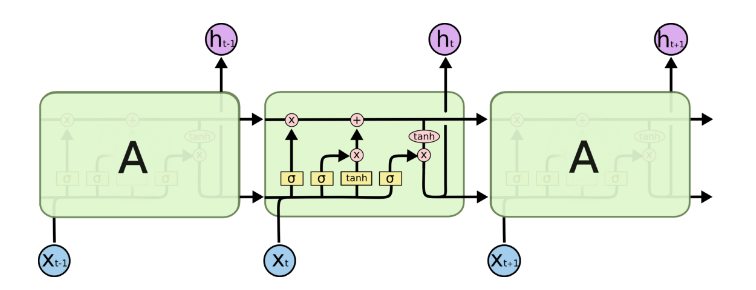LSTM的核心是细胞状态，用贯穿细胞的水平线表示。细胞状态像传送带一样。它贯穿整个细胞却只有很少的分支，这样能保证信息不变的流过整个结构。同时，LSTM通过称为门（gate）的结构来对单元状态进行增加或删除，包含三扇门：

• 遗忘门：决定哪些信息丢弃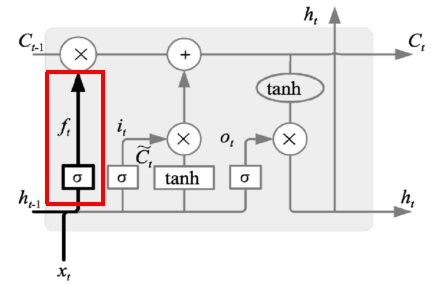表达式为：$f_t = \sigma (W_f \cdot [h_{t-1}, x_t] + b_f)$，当输出为1时表示完全保留，输出为0是表示完全丢弃

• 输入门：决定哪些信息输入进来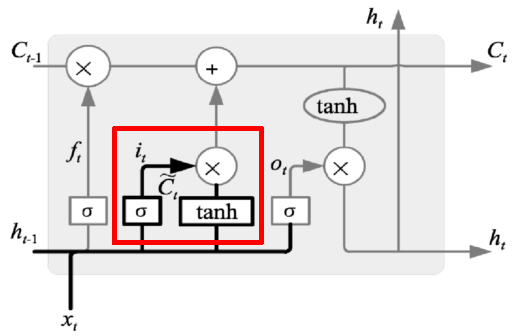表达式为：

$i_t = \sigma (W_i \cdot [h_{t-1}, x_t] + b_i) \\ \tilde{C}_t = tanh(W_c \cdot [h_{t-1}, x_t] + b_c)$

根据输入、遗忘门作用结果，可以对细胞状态进行更新，如下图所示：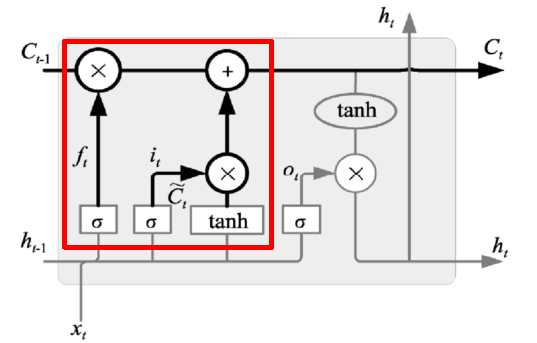状态更新表达式为：

$C_t = f_t \cdot C_{t-1} + i_t \cdot \tilde{C}_t$

遗忘门找到了需要忘掉的信息$f_t$后，再将它与旧状态相乘，丢弃掉确定需要丢弃的信息。再将结果加上$i_t \cdot C_t$使细胞状态获得新的信息，这样就完成了细胞状态的更新。

• 输出门：决定输出哪些信息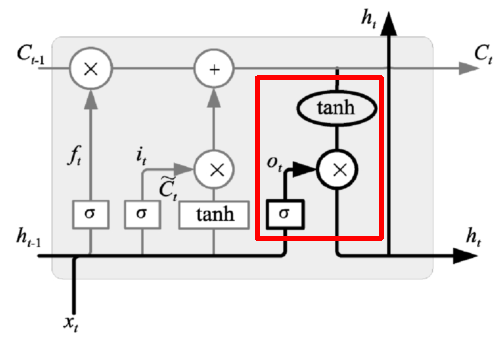$O_t = \sigma (W_o \cdot [h_{t-1}, x_t] + b_o) \\ h_t = O_t \cdot tanh(C_t)$

#### 3）双向循环神经网络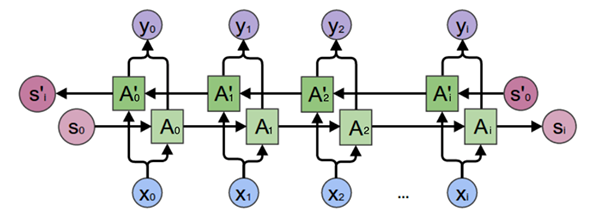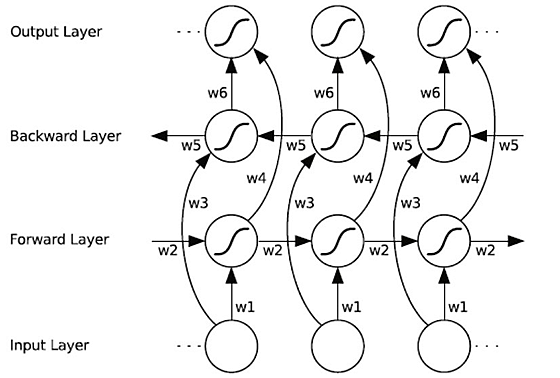$h_t = f(w_1x_t + w_2h_{t-1}) \\ h_t' = f(w_3x_t + w_5h'_{t+1}) \\ o_t = g(w_4h_t + w_6h'_t)$

## 五、NLP应用

### 1. 文本分类

#### 2）文本分类的应用

• 内容分类（新闻分类）
• 邮件过滤（例如垃圾邮件过滤）
• 用户分类（如商城消费级别、喜好）
• 评论、文章、对话的情感分类（正面、负面、中性）

#### 3）文本分类案例

• 任务：建立文本分类模型，并对模型进行训练、评估，从而实现对中文新闻摘要类别正确划分
• 数据集：从网站上爬取56821条数据中文新闻摘要，包含10种类别，国际、文化、娱乐、体育、财经、汽车、教育、科技、房产、证券，各类别样本数量如下表所示：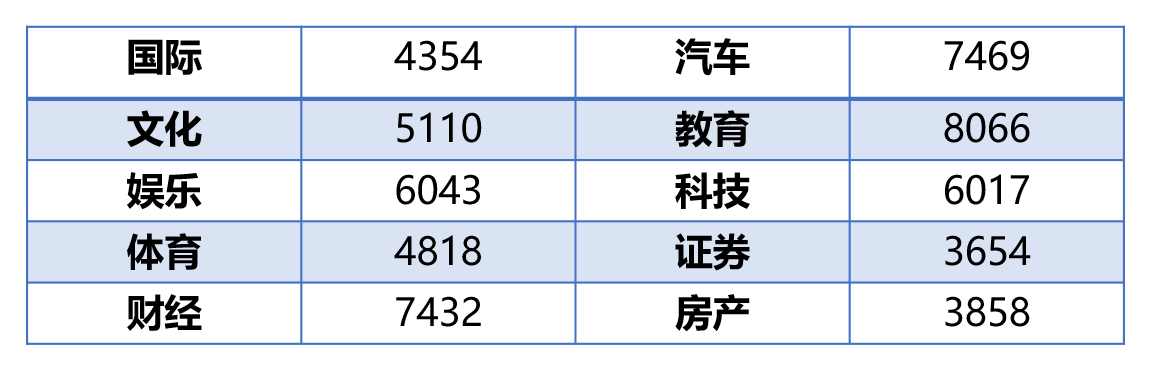• 模型选择：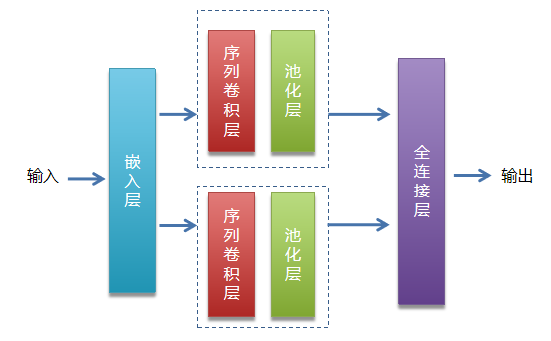• 步骤：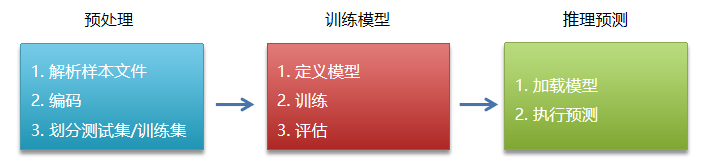• 代码

【预处理部分】

【模型定义与训练】

【推理预测】

### 2. 文本情感分析

1）目标：利用训练数据集，对模型训练，从而实现对中文评论语句情感分析。情绪分为正面、负面两种

2）数据集：中文关于酒店的评论，5265笔用户评论数据，其中2822笔正面评价、其余为负面评价

3）步骤：同上一案例

4）模型选择：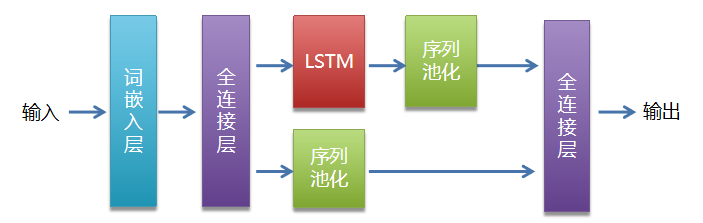5）代码

【数据预处理】

【模型定义与训练】

【推理预测】

## 附录一：相关数学知识

1）向量余弦相似度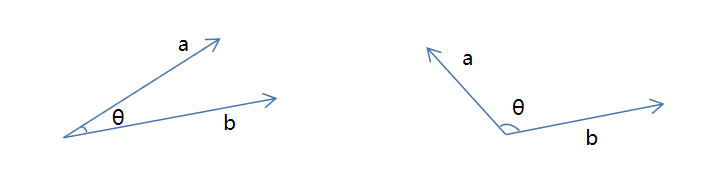$cos \theta = \frac{ab}{||a|| \times ||b||}$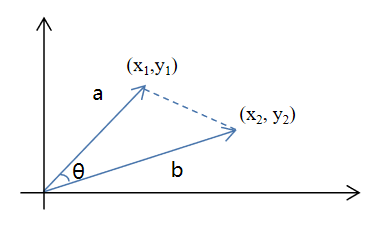$cos \theta = \frac{a^2 + b^2 - c^2}{2ab} \\ = \frac{\sqrt{x_1^2 + y_1^2} + \sqrt{x_2^2 + y_2^2 }+ \sqrt{(x_1 - x_2)^2 + (y_1 - y_2)^2}}{2 \sqrt{x_1^2 + y_1^2} \sqrt{x_2^2 + y_2^2}} \\ = \frac{2 x_1 x_2 + 2 y_1 y_2}{2 \sqrt{x_1^2 + y_1^2} \sqrt{x_2^2 + y_2^2}} = \frac{ab}{||a|| \times ||b||}$

## 附录二：参考文献

1）《Python自然语言处理实践——核心技术与算法》 ，涂铭、刘祥、刘树春 著 ，机械工业出版社

2）《Tensorflow自然语言处理》，【澳】图珊·加内格达拉，机械工业出版社

3）《深度学习之美》，张玉宏，中国工信出版集团 / 电子工业出版社

4）网络部分资源

## 附录三：专业词汇列表

NLP Nature Language Processing 自然语言处理
NER Named Entities Recognition 命名实体识别
PoS part-of-speech tagging 词性标记
MT Machine Translation 机器翻译
TF-IDF Term Frequency-Inverse Document Frequency 词频-逆文档频率
Text Rank 文本排名算法
One-hot 独热编码
BOW Bag-of-Words Model 词袋模型
N-Gram N元模型
word embedding 词嵌入
NNLM Neural Network Language Model 神经网络语言模型
HMM Hidden Markov Model 隐马尔可夫模型
RNN Recurrent Neural Networks 循环神经网络
Skip-gram 跳字模型
CBOW Continous Bag of Words 连续词袋模型
LSTM Long Short Term Memory 长短期记忆模型
GRU Gated Recurrent Unit 门控环单元
BRNN Bi-recurrent neural network 双向循环神经网络
FMM Forward Maximum Matching 正向最大匹配
RMM Reverse Maximum Matching 逆向最大匹配
Bi-MM Bi-directional Maximum Matching 双向最大匹配法